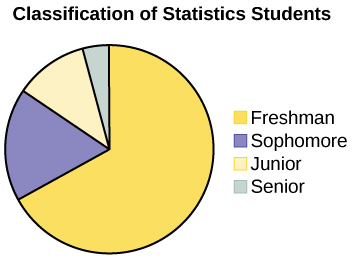# 3 1.2 Data: Quantitative Data & Qualitative Data

Data may come from a population or from a sample. Small letters like x or y generally are used to represent data values. Most data can be put into the following categories:

• Qualitative
• Quantitative
 Quantitative Data Qualitative Data Definition Quantitative data are the result of counting or measuring attributes of a population. Qualitative data are the result of categorizing or describing attributes of a population. Data that you will see Quantitative data are always numbers. Qualitative data are generally described by words or letters. Examples Amount of money you have Height Weight Number of people living in your town Number of students who take statistics Hair color Blood type Ethnic group The car a person drives The street a person lives on

Researchers often prefer to use quantitative data over qualitative data because it lends itself more easily to mathematical analysis. For example, it does not make sense to find an average hair color or blood type.

Quantitative data may be either discrete or continuous.

All data that are the result of counting are called quantitative discrete data. These data take on only certain numerical values. If you count the number of phone calls you receive for each day of the week, you might get values such as zero, one, two, or three.

All data that are the result of measuring are quantitative continuous data assuming that we can measure accurately. Measuring angles in radians might result in such numbers as $\displaystyle\frac{pi}{{6}},\frac{pi}{{3}},\frac{pi}{{2}},pi,\frac{{{3}pi}}{{4}}$ , and so on. If you and your friends carry backpacks with books in them to school, the numbers of books in the backpacks are discrete data and the weights of the backpacks are continuous data.

### Example of Quantitative Discrete Data

The data are the number of books students carry in their backpacks. You sample five students. Two students carry three books, one student carries four books, one student carries two books, and one student carries one book. The numbers of books (three, four, two, and one) are the quantitative discrete data.

### Try It

The data are the number of machines in a gym. You sample five gyms. One gym has 12 machines, one gym has 15 machines, one gym has ten machines, one gym has 22 machines, and the other gym has 20 machines. What type of data is this?

It is quantitative discrete data

### Example of Quantitative Continuous Data

The data are the weights of backpacks with books in them. You sample the same five students. The weights (in pounds) of their backpacks are 6.2, 7, 6.8, 9.1, 4.3. Notice that backpacks carrying three books can have different weights. Weights are quantitative continuous data because weights are measured.

### Try It

The data are the areas of lawns in square feet. You sample five houses. The areas of the lawns are 144 sq. feet, 160 sq. feet, 190 sq. feet, 180 sq. feet, and 210 sq. feet. What type of data is this?

It is quantitative continuous data.

### Examples of Qualitative Data

The data are the colors of backpacks. Again, you sample the same five students. One student has a red backpack, two students have black backpacks, one student has a green backpack, and one student has a gray backpack. The colors red, black, black, green, and gray are qualitative data.

### Try It

A statistics professor collects information about the classification of her students as freshmen, sophomores, juniors, or seniors. The data she collects are summarized in the pie chart.What type of data does this graph show?

This pie chart shows the students in each year, which is qualitative data.

## Example 1

Determine the correct data type (quantitative or qualitative). Indicate whether quantitative data are continuous or discrete. Hint: Data that are discrete often start with the words “the number of.”

1. The number of pairs of shoes you own
2. The type of car you drive
3. The place where you go on vacation
4. The distance it is from your home to the nearest grocery store
5. The number of classes you take per school year.
6. The tuition for your classes
7. The type of calculator you use
8. Movie ratings
9. Political party preferences
10. Weights of sumo wrestlers
11. Amount of money (in dollars) won playing poker
12. Number of correct answers on a quiz
13. Peoples’ attitudes toward the government
14. IQ scores

Items a, e, f, k, and l are quantitative discrete; items d, j, and n are quantitative continuous; items b, c, g, h, i, and m are qualitative.

## Omitting Categories and Missing Data

The table displays Ethnicity of Students but is missing the “Other/Unknown” category. This category contains people who did not feel they fit into any of the ethnicity categories or declined to respond. Notice that the frequencies do not add up to the total number of students. In this situation, create a bar graph and not a pie chart.

Frequency Percent
Asian 8,794 36.1%
Black 1,412 5.8%
Filipino 1,298 5.3%
Hispanic 4,180 17.1%
Native American 146 0.6%
Pacific Islander 236 1.0%
White 5,978 24.5%
TOTAL 22,044 out of 24,382 90.4% out of 100%Figure 1. Ethnicity of Students

The following graph is the same as the previous graph but the “Other/Unknown” percent (9.6%) has been included. The “Other/Unknown” category is large compared to some of the other categories (Native American, 0.6%, Pacific Islander 1.0%). This is important to know when we think about what the data are telling us.

This particular bar graph in Figure 2 can be difficult to understand visually.Figure 2. Bar Graph with Other/Unknown Category

The graph in Figure 3 is a Pareto chart. The Pareto chart has the bars sorted from largest to smallest and is easier to read and interpret.Figure 3. Pareto Chart with Bars Sorted by Size